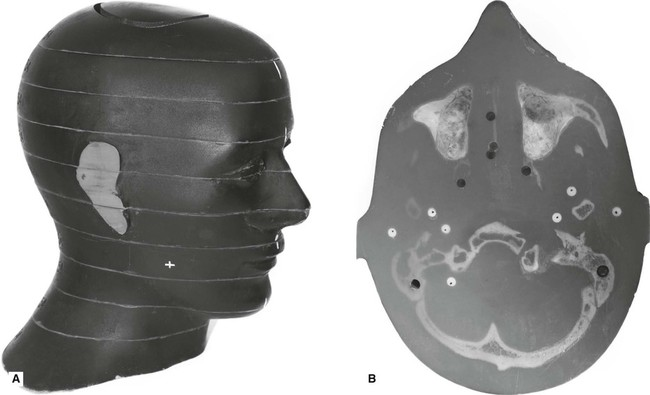# Radiation dose, dosimetry and dose limitation

Several different terms and units have been used in dosimetry over the years. The use of the word dose in multiple different descriptors has made this subject even more confusing. However, it is essential that these terms and units are understood to appreciate what is meant by radiation dose and to allow meaningful comparisons between different investigations to be made. In addition to explaining the various units and how they are measured/calculated, this chapter also covers the concept of clinical dose limits and dose limitation, as well as summarizing the estimated doses from various sources of ionizing radiation and the magnitude of radiation doses from common dental and medical clinical investigations.

The more important terms in dosimetry include:

## Dose units

This is a measure of the amount of energy absorbed from the radiation beam per unit mass of tissue and can be measured using a dosimeter.

 SI unit: joules/kg (J/kg) Special name: Gray (Gy) Subunit names: milligray (mGy) (× 10−3) microgray (µGy) (× 10−6)

### Equivalent dose (HT)

This is a measure which allows the different radiobiological effectiveness (RBE) of different types of radiation to be taken into account.

For example, alpha particles (see Ch. 18) penetrate only a few millimeters in tissue, lose all their energy and are totally absorbed, whereas X-rays penetrate much further, lose some of their energy and are only partially absorbed. The biological effect of a particular radiation-absorbed dose of alpha particles would be considerably more severe than a similar radiation-absorbed dose of X-rays.

By introducing a numerical value known as the radiation weighting factor (WR) which represents the biological effects of different radiations on different tissues, the unit of equivalent dose (HT) in a particular tissue provides a common unit allowing comparisons to be made between one type of radiation and another, for example:

 X-rays, gamma rays and beta particles WR = 1 Fast neutrons (10 keV – 100 keV) and protons WR = 10 Alpha particles WR = 20

The equivalent dose (HT) in a particular tissue is therefore calculated as follows:Equivalent dose (HT) = radiation-absorbed dose (D) × radiation weighting factor (WR) in a particular tissue

 SI unit: joules/kg (J/kg) Special name: Sievert (Sv) Subunit names: millisievert (mSv) (× 10−3) microsievert (µSv) (× 10−6)

For X-rays, the radiation weighting factor WR = 1, therefore the equivalent dose (HT) in a particular tissue, measured in Sieverts, is equal to the radiation-absorbed dose (D), measured in Grays.

### Effective dose (E)

This measure allows doses from different investigations of different parts of the body to be compared, by converting all doses to an equivalent whole body dose. This is necessary because some parts of the body are more sensitive to radiation than others. The International Commission on Radiological Protection (ICRP) has allocated each tissue a numerical value, known as the tissue weighting factor (WT), based on its radiosensitivity, i.e. the risk of the tissue being damaged by radiation – the greater the risk, the higher the tissue weighting factor. The sum of the individual tissue weighting factors represents the weighting factor for the whole body.

Table 6.1

The tissue weighting factors (WT) recommended by the ICRP in 1990 and revised in 2007

 Tissue 1990 WT 2007 WT Bone marrow 0.12 0.12 Breast 0.05 0.12 Colon 0.12 0.12 Lung 0.12 0.12 Stomach 0.12 0.12 Gonads 0.20 0.08 Bladder 0.05 0.04 Oesophagus 0.05 0.05 Liver 0.05 0.04 Thyroid 0.05 0.04 Bone surface 0.01 0.01 Brain * 0.01 Kidneys * 0.01 Salivary glands – 0.01 Skin 0.01 0.01 Remainder tissues 0.05* 0.12†

The sum of the individual tissue weighting factors represents the weighting factor for the whole body. The effective dose (E) from an individual clinical radiograph, where the X-ray beam is absorbed by different tissues, is calculated as follows:

Effective dose (E) = ∑ Equivalent dose (HT) in each tissue × relevant tissue weighting factor (WT)

 Special name: sievert (Sv) Subunit names: millisievert (mSv) (× 10−3) microsievert (µSv) (× 10−6)

The 2007 revised tissue weighting factors include the salivary glands as an individual weighted tissue and the oral mucosa in the remainder tissues. Therefore the effective dose for dental radiographic examinations may be considerably higher when calculated using these revised tissue weighting factors.Fig. 6.1 A An example of an anthropomorphic phantom used in dosimetry. B One slice of the phantom showing the positions where the individual dosimeters can be placed in specific radiosensitive tissues.

As individual doses are very small from any one examination, a number of radiographic exposures are repeatedly performed (e.g. ten times) using typical exposure factors (time, kV and mA), for that particular examination. The dosimeters are then ‘read’ to give a measurement of the radiation-absorbed dose (D) in the individual tissues. Using this data both the equivalent dose (HT) and the effective dose (E)

Feb 12, 2015 | Posted by in Oral and Maxillofacial Radiology | Comments Off on Radiation dose, dosimetry and dose limitation
Premium Wordpress Themes by UFO Themes